# Coherent Detection

It is clear from the discussion of optical receiver noise tutorial that, even though shot noise sets the fundamental limit, it is the thermal noise that limits a photodetector in practice. The use of APDs helps to reduce the impact of thermal noise to some extent, but it also enhances the shot noise. One may ask if it is possible to design a detection scheme that is limited by shot-noise alone. The answer is provided by a technique known as coherent detection, so called because it combines the incoming optical signal coherently with a CW optical field before it falls on the detector. An added benefit is that such a technique can also be used for systems that encode information in the optical phase (such as FSK and PSK modulation formats) because it converts phase variations into amplitude variations.

#### 1. Local Oscillator

The basic idea behind coherent detection is shown schematically in the figure below.A coherent field is generated locally at the receiver using a narrow-linewidth laser, called the local oscillator (LO), a term borrowed from the radio and microwave literature.  It is combined with the incoming optical field using a beam splitter, typically a fiber coupler in practice. To see how such a mixing can improve the receiver performance, let us write the optical signal using complex notation as

Es = Asexp[-i(ω0t + φs)]

where ω0 is the carrier frequency, As is the amplitude, and φs is the phase. The optical field associated with the local oscillator is given by a similar expression,

ELO = ALOexp[-i(ωLOt + φLO)]

where ALOωLO, and φLO represent the amplitude, frequency, and phase of the local oscillator, respectively. The scalar notion is used for both Es and ELO after assuming that the two fields are identically polarized. The optical power incident at the photodetector is given by P = |Es + ELO|2. Using the two equations above,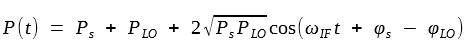where

Ps = As2,  PLO = ALO2, ωIF = ω0 - ωLO

The frequency νIF ≡ ωIF/2π is known as the intermediate frequency (IF). When ω0 ≠ ωLO, the optical signal is demodulated in two stages. Its carrier frequency is first converted to an intermediate frequency νIF (typically 0.1-5 GHz). The resulting radio-frequency (RF) signal is then processed electronically to recover the bit stream. It is not always necessary to use an intermediate frequency. In fact, there are two different coherent detection techniques to choose from, depending on whether or not ωIF equals zero. They are known as homodyne and heterodyne detection techniques.

#### 2. Homodyne Detection

In this coherent-detection technique, the local-oscillator frequency ωLO is selected to coincide with the signal-carrier frequency ω0 so that ωIF = 0. From the equation above, the photocurrent (I = RdP, where Rd is the detector responsivity) is given by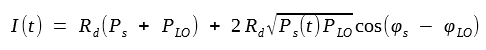Typically, PLO >> Ps, and Ps + PLO ≈ PLO. The last term in this equation contains the information transmitted and is used by the decision circuit. Consider the case in which the local-oscillator phase is locked to the signal phase so that φs = φLO. The homodyne signal is then given byThe main advantage of homodyne detection is evident from this equation if we note that the signal current in the direct-detection case is given by Idd(t) = RdPs(t). Denoting the average signal power by, the average electrical power is increased by a factor of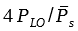with the use of homodyne detection. Since PLO can be made much larger than, the power enhancement can exceed 20 dB. Although shot noise is also enhanced, it is shown later that homodyne detection improves the SNR by a large factor.

Another advantage of  coherent detection is evident from the I(t) equation above. Because the last term in this equation contains the signal phase explicitly, it is possible to recover data transmitted using the phase or frequency of the optical carrier. Direct detection does not allow this because all information in the signal phase is lost. Several modulation formats requiring phase encoding are discussed in another tutorial.

A disadvantage of homodyne detection also results from its phase sensitivity. Since the last term in I(t) equation above contains the local-oscillator phase φLO explicitly, clearly φLO should be controlled. Ideally, φs and φLO should stay constant except for the intentional modulation of φs. In practice, both φs and φLO fluctuate with time in a random manner. However, their difference φs - φLO can be forced to remain nearly constant through an optical phase-locked loop. The implementation of such a loop is not simple and makes the design of optical homodyne receivers quite complicated. In addition, matching of the transmitter and local-oscillator frequencies puts stringent requirements on the two optical sources.

#### 3. Heterodyne Detection

In the case of heterodyne detection the local-oscillator frequency ωLO is chosen to differ from the signal-carrier frequency ω0 such that the intermediate frequency ωIF is in the microwave region (νIF ~ 1 GHz).  Using the P(t) equation from above together with I = RdP, the photocurrent is now given bySince PLO >> Ps in practice, the direct-current (dc) term is nearly constant and can be removed easily using bandpass filters. The heterodyne signal is then given by the alternating-current (ac) term in this equation or bySimilar to the case of homodyne detection, information can be transmitted through amplitude, phase, or frequency modulation of the optical carrier. More importantly, the local oscillator still amplifies the received signal by a large factor, thereby improving the SNR. However, the SNR improvement is lower by a factor of 2 (or by 3 dB) compared with the homodyne case. This reduction is referred to as the heterodyne detection penalty. The origin of the 3-dB penalty can be seen by considering the signal power (proportional to the square of the current). Because of the ac nature of Iac, the electrical power is reduced by a factor of 2 when Iac2 is averaged over a full cycle at the intermediate frequency (recall that the average of cos2θ over θ is 1/2).

The advantage gained at the expense of the 3-dB penalty is that the receiver design is simplified considerably because an optical phase-locked loop is no longer needed. Fluctuations in both φs and φLO still need to be controlled using narrow-linewidth semiconductor lasers for both optical sources. However, the linewidth requirements are relatively moderate when an asynchronous demodulation scheme is employed. This feature makes the heterodyne-detection scheme quite suitable for practical implementation in coherent lightwave systems.

#### 4. Signal-to-Noise Ratio

The advantage of coherent detection for lightwave systems can be made more quantitative by considering the SNR of the receiver current. For this purpose, it is necessary to extend the analysis of optical receiver noise to the case of heterodyne detection. The receiver current fluctuates because of shot noise and thermal noise. The variance σ2 of current fluctuations is obtained by adding the two contributions so that

σ2 = σs2 + σT2

where

σs2 = 2q(I + Id)Δf,   σT2 = (4kBT/RL)FnΔf

It is important to note that the I in this equation is the total current generated at the detector and is given by I(t) from the two equations above, depending on whether homodyne or heterodyne detection is employed. In practice, PLO >> Ps, and I in this equation can be replaced by the dominant term RPLO for both cases.

The SNR is obtained by dividing the average signal power by the average noise power. In the heterodyne case, it is given by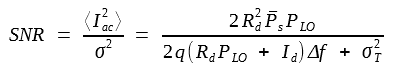In the homodyne case, the SNR is larger by a factor of 2 if we assume that φs = φLO. The main advantage of coherent detection can be seen from this equation. Since the local-oscillator power PLO can be controlled at the receiver, it can be made large enough that the receiver noise is dominated by shot noise. More specifically, σs2 >> σT2 when

PLO >>  σT2 /(2qRdΔf)

Under the same conditions, the dark-current contribution to the shot noise is negligible (Id <<  RPLO). The SNR is then given by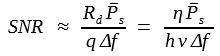where Rd = ηq/hν was used. The main point to emphasize is that the use of coherent detection allows one to achieve the shot-noise limit even for p-i-n receivers whose performance is generally limited by thermal noise. Moreover, in contrast with the case of APDs, this limit is realized without adding any excess shot noise.

It is useful to express the SNR in terms of the number of photons, Np, received within a single bit. At the bit rate B, the signal power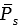is related to Np as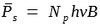. Typically, Δf ≈ B/2. Using these values in the SNR equation above, the SNR is given by a simple expression

SNR = 2ηNp

In the case of homodyne detection, SNR is larger by a factor of 2 and is given by

SNR = 4ηNp